# Motion2

Cyclist started out of town at 19 km/h. After 0.7 hours car started behind him in the same direction and caught up with him for 23 minutes. How fast and how long went car from the city to caught cyclist?

Correct result:

v2 =  53.7 km/h
s =  20.6 km

#### Solution:

s = 19 t
s = v • 23/60
t = 0.7+23/60

s = 19•t
s = v • 23/60
t = 0.7+23/60

s-19t = 0
60s-23v = 0
60t = 65

s = 24712 ≈ 20.583333
t = 1312 ≈ 1.083333
v = 123523 ≈ 53.695652

Calculated by our linear equations calculator.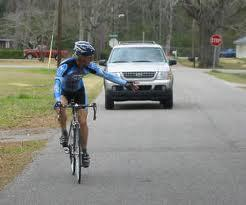Our examples were largely sent or created by pupils and students themselves. Therefore, we would be pleased if you could send us any errors you found, spelling mistakes, or rephasing the example. Thank you!

Please write to us with your comment on the math problem or ask something. Thank you for helping each other - students, teachers, parents, and problem authors.Tips to related online calculators
Need help calculate sum, simplify or multiply fractions? Try our fraction calculator.
Do you have a linear equation or system of equations and looking for its solution? Or do you have quadratic equation?
Do you want to convert length units?
Do you want to convert velocity (speed) units?
Do you want to convert time units like minutes to seconds?

## Next similar math problems:

• The tourist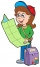The tourist walked a quarter of the way on the first day, a third of the rest on the second day, and 20 km on the last day. How many km did he walked in three days?
• Double ratio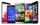The mobile phone was twice gradually discounted in the ratio of 3: 2 1 half: 5 quarters. How much did it originally cost if the price was CZK 4,200 after a double discount?
• How many 3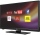How many hours the Andersons watched TV in all Wednesday 3/1 hr Thursdays 2/3 hr Friday 4/5 hr Saturday 3/4 hr
• What time is it?What time is it, when there is 4 times less time left until midnight than the time that has elapsed since noon? What time is it, when one-fifth of the hours that have passed since midnight is equal to one-third of the hours that are missing by 12 o'clock?
• Pizza palaceJosh is at Enzo's pizza palace. He can sit at a table with 5 of his friends or at a different table with seven of his friends. The same size pizza is shared equally among the people at each table. At which table should Josh sit to get more pizza? (write a
• CoinsThe money - coins are minted from the hardest bronze, which contains copper and tin in a ratio of 41: 9. How much copper and tin are in 2kg of bronze money?
• Two ribbonsThe total length of two ribbons is 13 meters. If one ribbon is 7 and 5/8 meters long, what is the length of the other ribbon.2 and 1 8th plus 1 and 1 3rd =
• Mixing 5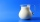Carlos mixed 4/15 of chocolate syrup with 1/2 of milk. Determine the reasonable estimate of the total amount of liquid
• Equilateral coneWe pour so much water into a container that has the shape of an equilateral cone, the base of which has a radius r = 6 cm, that one-third of the volume of the cone is filled. How high will the water reach if we turn the cone upside down?
• Final exam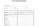At the final exam, the student answers from three areas, which are evaluated in a ratio of 1: 2: 2. What grade will John receive if he answered as follows: 3,1,2.
• The plan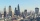The plan of the housing estate is in three scales 1: 5000,1: 10000,1: 15000. The distance between two points on a plan with a scale of 1: 10000 is 12 cm. What is this distance on the other two plans? What is this distance?How many times does the surface of a sphere decrease if we reduce its radius twice?Line segment AB 12 cm long divide in a ratio of 5: 3. How long are the individual parts?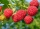Alena collected 7.8 kg of blueberries, 2.6 kg of blackberries, and 3.9 kg of cranberries. Express the ratio in the smallest natural numbers in this order.We want to split the number 110 into three summands so that the first and the second summand are in the ratio 4: 5, and the third with the first are in ratio 7: 3. Calculate the smallest of the summands.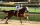A farmer died leaving his 17 horses to his 3 sons. When his sons opened up the Will it read: My eldest son should get 1/2 (half) of total horses; My middle son should be given 1/3rd (one-third) of the total horses; My youngest son should be given 1/9th (